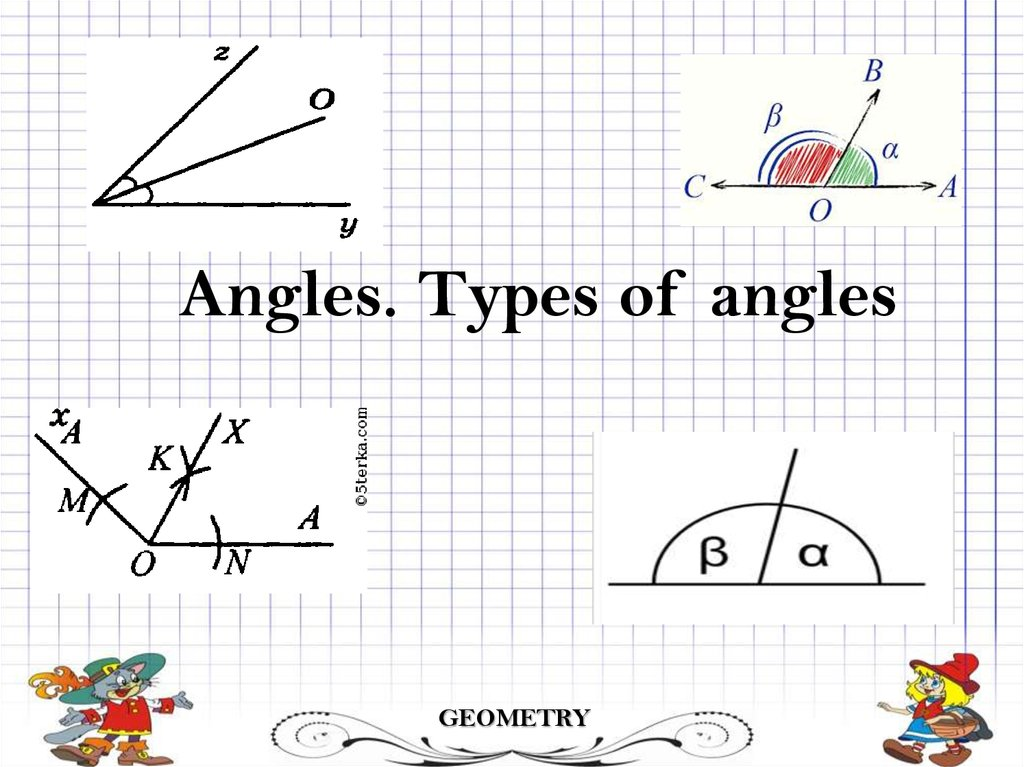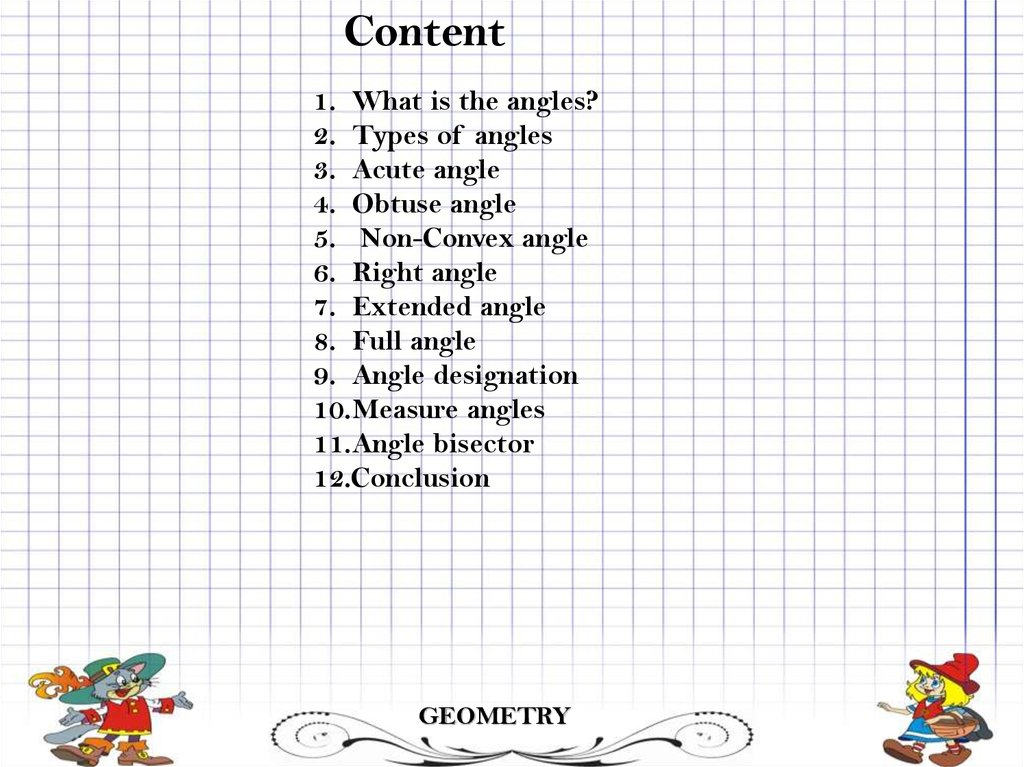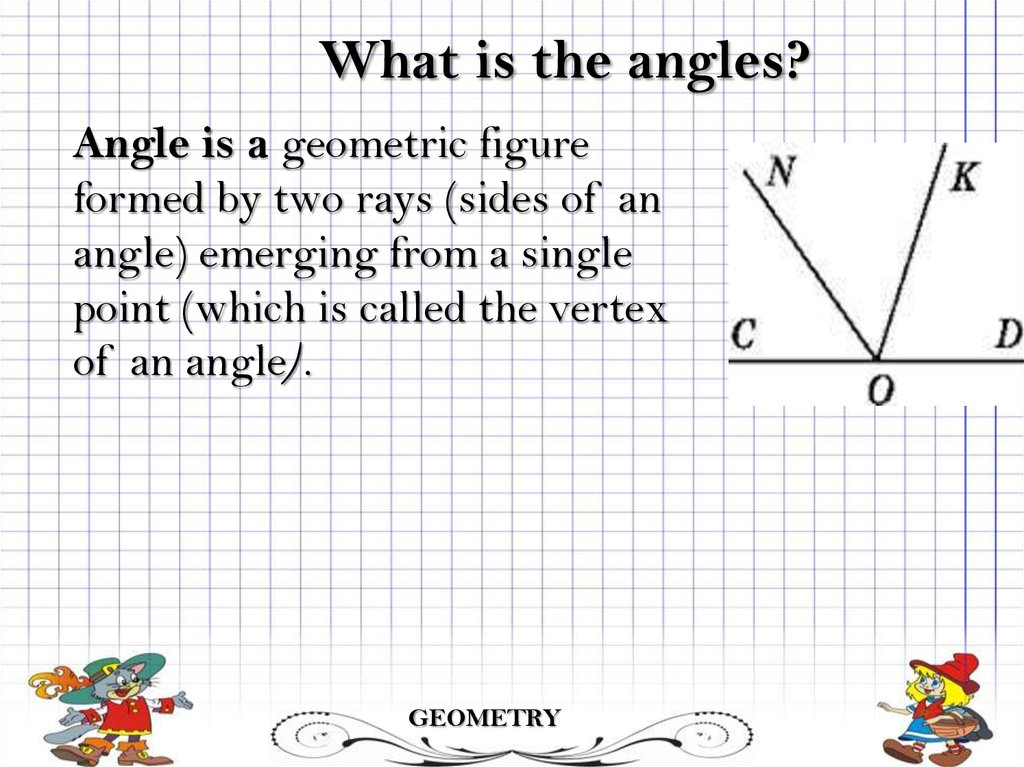# Angles. Types of angles

## 1.

Angles. Types of angles
GEOMETRY

## 2.

Content
1. What is the angles?
2. Types of angles
3. Acute angle
4. Obtuse angle
5. Non-Convex angle
6. Right angle
7. Extended angle
8. Full angle
9. Angle designation
10.Measure angles
11.Angle bisector
12.Conclusion
GEOMETRY

## 3.

What is the angles?
Angle is a geometric figure
formed by two rays (sides of an
angle) emerging from a single
point (which is called the vertex
of an angle).
GEOMETRY

## 4.

Types of angles
•Acute angles (0-90)
•Obtuse angles(90-180)
•Non-convex angles(180360)
•Right angles(90)
•Straight angles(180)
•Full angles (360)
GEOMETRY

## 5.

Acute angle
The measure of an angle lies
between 0 and 90 or with less
than 90 is called as acute angle
Also: the letter "A" has an acute angle
GEOMETRY

## 6.

Obtuse angle
An Obtuse Angle is more than 90° but less
than 180°
A triangle in which one of the angles
is an obtuse angle, i.e., more than 90
(other two angles are acute) is called
an obtuse-angled triangle or obtuse
triangle.
GEOMETRY

## 7.

Non-convex angle
A Non-convex angle is more
than 180° but less than 360
The non-convex angel is the larger
angle, an unbounded geometric figure
formed by two rays (sides of an angle)
emerging from a single point (the angle
vertex).
GEOMETRY

## 8.

Straight angles
A straight angle is 180 degrees.
A straight angle changes the
direction to point the opposite way.
Sometimes people say "You did a
complete 180 on that!" ...
meaning you completely changed
GEOMETRY

## 9.

Full angle
The circle will be * 360 = 1 ° *
360 = 360 °.
The angle equal to the plane of
the circle is 360 ° and is called
the full angle
GEOMETRY
GEOMETRY

## 10.

Bisector of angle
The bisector (from Latin bi"double", and sectio "cutting")
of the angle is a ray emanating
from the top of the corner and
dividing the angle into two
equal angles. One can also define
a bisectrix as the locus of points
inside an angle equidistant from
the sides of this angle.
GEOMETRY

## 11.

Measure of angle
A degree is called 1/180 part of
the unfolded angle. Indicate 1 °.
Degree of angle is measured by
protractor
Degree measure of an angle is a
number that indicates how many
times it contains a unit of degree
measurement
GEOMETRY

## 12.

Angel designation
The sign for angles was first introduced in the
18th century by the French mathematician
Erigon Pierre
Erigon used a sign to denote a right
angle
The first designation of the angle was invented by
the French mathematician Erigon Pierre
GEOMETRY

## 13.

Conclusion
1.Angle is a geometric figure formed
by two rays
2.Type of angles: acute angle, obtuse
angle, non-convex angle, right angles,
extended angle and full angles.
3. Each corner has its own degree
measure
4. Тhe first designation of the angle was
invented by the French
mathematician Erigon Pierre
Geometry